## The time , in hours , it takes to repair an electrical breakdown in a certain factory is represent by a random variable x where repair time

Question

The time , in hours , it takes to repair an electrical breakdown in a certain factory is represent by a random variable x where repair time follow a normal distribution with mean 5 hour and standard deviation 1 hour , if 5 electrical breakdown ore randomly chosen , Find the probability that ( a all repaired time below 6 hours ( b ) exactly 3 of them repaired below 6 hours

in progress 0
5 months 2021-09-01T03:17:58+00:00 1 Answers 3 views 0

a) 0.4215 = 42.15% probability that all are repaired in less than 6 hours.

b) 0.15 = 15% probability that exactly 3 of them repaired in a time below 6 hours

Step-by-step explanation:

To solve this question, we need to understand the normal and the binomial probability distributions.

Binomial probability distribution

The binomial probability is the probability of exactly x successes on n repeated trials, and X can only have two outcomes.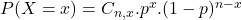In which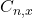is the number of different combinations of x objects from a set of n elements, given by the following formula.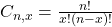And p is the probability of X happening.

Normal Probability Distribution:

Problems of normal distributions can be solved using the z-score formula.

In a set with mean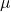and standard deviation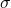, the z-score of a measure X is given by: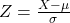The Z-score measures how many standard deviations the measure is from the mean. After finding the Z-score, we look at the z-score table and find the p-value associated with this z-score. This p-value is the probability that the value of the measure is smaller than X, that is, the percentile of X. Subtracting 1 by the p-value, we get the probability that the value of the measure is greater than X.

Proportion repaired below 6 hours:

Mean 5 hours and standard deviation 1 hour, which means that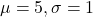This proportion is the p-value of Z when X = 6. So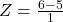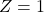has a p-value of 0.8413.

0.8413 repaired below 6 hours.

For the binomial distribution, this means that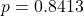5 are chosen:

This means that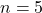a) Probability that all are repaired in less than 6 hours

This is P(X = 5). So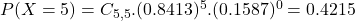0.4215 = 42.15% probability that all are repaired in less than 6 hours.

b) Probability that exactly 3 of them repaired below 6 hours

This is P(X = 3). So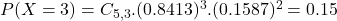0.15 = 15% probability that exactly 3 of them repaired in a time below 6 hours